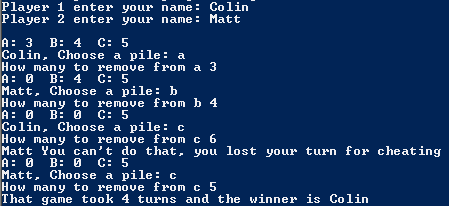# Project #2

## Code

```///colin hinton
///5th period
///Nim
///Nim.java
///11/30/2015

import java.util.Scanner;

class Nim
{
public static void main (String [] args)
{
Scanner keyboard = new Scanner(System.in);

String nameOne, nameTwo, choice;
int take, a, b, c, turn;
a = 3;
b = 4;
c = 5;
turn = 0;
System.out.print("Player 1 enter your name: ");
nameOne = keyboard.next();
System.out.print("Player 2 enter your name: ");
nameTwo = keyboard.next();
System.out.println();
while ( (a + b + c) != 0 )
{
System.out.println("A: " + a + "  B: " + b + "  C: " + c);
if (turn%2 == 0)
{
turn++;
System.out.print(nameOne + ", Choose a pile: ");
}
else
{
turn++;
System.out.print(nameTwo + ", Choose a pile: ");
}
choice = keyboard.next();
System.out.print("How many to remove from " + choice + " ");
take = keyboard.nextInt();
if (choice.equals("a") && ( a != 0) && ((a - take) >= 0 ) && (take >= 1 ))
{
a = (a - take);
}
else if (choice.equals("b") && ( b != 0) && ((b - take) >= 0 ) && (take >= 1 ))
{
b = (b - take);
}
else if (choice.equals("c") && ( c != 0) && ((c - take) >= 0 ) && (take >= 1 ))
{
c = (c - take);
}
else
{
if (turn%2 == 0)
{
System.out.println(nameOne + " You can't do that, you lost your turn for cheating");
}
else
{
System.out.println(nameTwo + " You can't do that, you lost your turn for cheating");
}
}
}

if (turn%2 == 0)
{
System.out.println("That game took "+ turn + " turns and the winner is "+ nameOne);
}
else
{
System.out.println("That game took "+ turn + " turns and the winner is "+ nameTwo);
}
}
}

```

### Picture of the output